# StarForce C++ Obfuscator

This efficient solution is designed to obfuscate (transform) the source code of C/C++programs (supports standards C++98, C++03, C++11, C++14, C++17) to protect them against reverse engineering. After obfuscation the code is reliably protected against analysis that can be performed by a man or machine.StarForce C++ Obfuscator is recommended for the cases when software protection against reverse engineering is crucial. For example, it is vital for software that contains DRM keys or other sensitive data that can’t be lost or modified. If it happens it causes financial and reputation losses.

## There are two approaches to code obfuscationSource code obfuscation Code obfuscation with the program logic changing The program removes comments, spaces, joining all lines, replaces symbols, numeric constants and characters in strings with something more difficult for understanding but it doesn’t change the logic of obfuscated program. It means that binary codes for both a not obfuscated text and an obfuscated text will look the same. Developers may use this type of obfuscation when they have to supply the source code according to the license agreement. In this case, extra branching, loops, function calls, etc. are added. Binary codes for a not obfuscated text and an obfuscated text will be very different. Each obfuscation gives a new version of the binary file. For example, in order to stop working existing cheats and bots in the game, it's enough to re-obfuscate the source code without changing anything inside. This option provides the highest level of protection from analysis and modification.

StarForce C++ Obfuscator belongs to the second type. It involves different algorithms to change logic of the programm. At the moment it is one of the best products to protect С and C++ source code against cracking thanks to an optimal selection of protection methods and many years of work without compromising.

One of the StarForce C++ Obfuscator’s benefits is its versatility as it can be applied to protect programs designed for any operating systems and any processors, including protection of firmware.

## Sample of obfuscation

Source code before obfuscation Source code after obfuscation
```#include <stdio.h>

// Euclidian algorithm for calculating greatest common divisor (before obfuscation)
int gcd( int n, int m )
{
if( n < 1 || m < 1 ) return -1;
while( n != m )
{
if( n > m ) n -= m;
else m -= n;
}
return n;
}

// Tests in triplets { n, m, expected_gcd( n, m ) }
int tests[][ 3 ] = {
{ 1, 2, 1 },
{ 3, 3, 3 },
{ 42, 56, 14 },
{ 249084, 261183, 111 },
};

// Perform tests
int main( int, char*[] )
{
printf( "Performing tests of gcd function:\n" );
bool passed = true;
for( int i = 0; i < sizeof( tests ) / sizeof( tests[ 0 ] ); i++ )
{
int n = tests[ i ][ 0 ];
int m = tests[ i ][ 1 ];
int expected_gcd = tests[ i ][ 2 ];
int calculated_gcd = gcd( n, m );
printf( "  %d. gcd( %d, %d ) = %d, ", i + 1, n, m, calculated_gcd );
if( calculated_gcd == expected_gcd )
{
printf( "OK.\n" );
}
else
{
printf( "error.\n" );
passed = false;
}
}
printf( "Tests %s.\n", passed ? "passed" : "failed" );
return passed ? 0 : 1;
}
```
```#include <stdio.h>

// Euclidian algorithm for calculating greatest common divisor (after obfuscation)
int gcd( int n_0, int m_1 )
{
bool temp_12;
unsigned int temp_13;
unsigned int temp_14;
unsigned int temp_15;
unsigned int temp_17;
int temp_26;
int temp_35;
bool temp_36;
unsigned int temp_37;
bool temp_38;
int temp_39;
int temp_40;
int temp_41;
int temp_42;
bool state0_43;
bool state1_44;
bool state2_45;
bool state3_46;
bool state4_47;
bool state5_48;
bool state6_49;
bool state7_50;

L1:
L0:
state0_43 = (bool)1;
state1_44 = (bool)state0_43;
state2_45 = (bool)state1_44;
state3_46 = (bool)state2_45;
goto L126;

L6:
temp_37 = ( unsigned int )(temp_17);
temp_38 = ( bool )( temp_36 == 0 );
if (state0_43) goto L158; else goto L40;

L14:
temp_41 = ( int )(temp_17);
temp_38 = ( bool )( temp_36 == 0 );
if (state0_43) goto L160; else goto L128;

L22:
temp_26 = ( int )(temp_41);
if (state4_47) goto L70; else goto L72;

L24:
temp_41 = (int)state6_49;
temp_42 = temp_41 - temp_40;
temp_26 = (int)temp_42;
temp_35 = temp_39 + temp_42;
temp_39 = (int)temp_35;
if (state1_44) goto L30; else goto L26;

L26:
temp_42 = ( int )( state0_43 == 0 );
temp_26 = temp_42 - temp_39;
temp_41 = (int)temp_26;
temp_35 = temp_40 + temp_26;
temp_40 = (int)temp_35;
goto L30;

L30:
temp_36 = temp_39 != temp_40;
if (temp_36) goto L22; else goto L82;

L38:
state1_44 = ( bool )( state5_48 == 0 );
temp_26 = temp_39 + temp_35;
temp_42 = ( int )( state5_48 == 0 );
temp_42 = temp_42 - temp_35;
temp_40 = temp_26 + temp_42;
temp_41 = (int)1495809726u;
// The next string is really just an assignment on 32bit platform
temp_41 = ( int )( ( size_t )( temp_41 ) + ( ( ( size_t )( temp_41 ) << 31 ) << 1 ) + ( ( ( ( size_t )( temp_41 ) << 31 ) << 1 ) >> 15 ) );
goto L44;

L40:
goto L128;

L42:
if (state3_46) goto L82; else goto L84;

L44:
temp_35 = temp_40 ^ temp_41;
temp_42 = (int)1495809726u;
if (state1_44) goto L6; else goto L100;

L46:
temp_41 = ( int )( state4_47 == 0 );
if (state3_46) goto L22; else goto L98;

L48:
// The next string is really just an assignment on 32bit platform
temp_41 = ( int )( ( size_t )( temp_41 ) + ( ( ( size_t )( temp_41 ) << 31 ) << 1 ) + ( ( ( ( size_t )( temp_41 ) << 31 ) << 1 ) >> 15 ) );
if (state7_50) goto L66; else goto L96;

L52:
temp_35 = temp_41 + temp_42;
if (state2_45) goto L76; else goto L94;

L54:
// The next string is really just an assignment on 32bit platform
temp_26 = ( int )( ( size_t )( temp_26 ) + ( ( ( size_t )( temp_26 ) << 31 ) << 1 ) + ( ( ( ( size_t )( temp_26 ) << 31 ) << 1 ) >> 15 ) );
if (state2_45) goto L14; else goto L102;

L56:
temp_36 = temp_39 <= temp_41;
temp_26 = ( int )(temp_17);
if (state5_48) goto L80; else goto L92;

L58:
temp_37 = (unsigned int)state6_49;
temp_42 = ( int )(temp_38);
if (state2_45) goto L48; else goto L90;

L60:
state2_45 = ( bool )( state6_49 == 0 );
temp_38 = (bool)0u;
if (temp_36) goto L74; else goto L146;

L62:
temp_35 = (int)0;
if (state3_46) goto L26; else goto L104;

L64:
temp_41 = ( int )(temp_17);
state3_46 = ( bool )( state6_49 == 0 );
state4_47 = ( bool )( state6_49 == 0 );
temp_35 = (int)state6_49;
temp_36 = temp_40 <= temp_35;
if (state0_43) goto L162; else goto L154;

L66:
state7_50 = (bool)state6_49;
state5_48 = (bool)state2_45;
temp_39 = (int)-1;
temp_40 = (int)1495809726u;
if (state6_49) goto L22; else goto L42;

L68:
temp_39 = (int)-1;
if (state7_50) goto L88; else goto L86;

L70:
temp_36 = temp_39 > temp_40;
temp_17 = ( unsigned int )(temp_36);
if (temp_36) goto L24; else goto L62;

L72:
temp_42 = temp_35 | temp_26;
temp_41 = temp_35 & temp_26;
temp_17 = ( unsigned int )(temp_42);
temp_37 = ( unsigned int )(temp_41);
temp_38 = temp_17 < temp_37;
if (temp_38) goto L154; else goto L148;

L74:
temp_35 = temp_39 - temp_40;
if (state3_46) goto L106; else goto L14;

L76:
temp_41 = ( int )( state0_43 == 0 );
temp_26 = temp_41 - temp_39;
temp_35 = temp_40 + temp_26;
temp_40 = (int)temp_35;
temp_36 = temp_39 != temp_40;
if (state2_45) goto L154; else goto L40;

L80:
temp_42 = temp_39 + temp_35;
temp_26 = (int)temp_42;
temp_40 = temp_42 - temp_35;
temp_13 = ( unsigned int )(state7_50);
temp_14 = (unsigned int)131u;
temp_13 = temp_13 * temp_14;
temp_14 = (unsigned int)1495809857u;
temp_13 = temp_14 - temp_13;
temp_41 = ( int )( ( ptrdiff_t )( ( temp_13 ) & 0xFFFFFFFF ) );
// The next string is really just an assignment on 32bit platform
temp_41 = ( int )( ( size_t )( temp_41 ) + ( ( ( size_t )( temp_41 ) << 31 ) << 1 ) + ( ( ( ( size_t )( temp_41 ) << 31 ) << 1 ) >> 15 ) );
if (state3_46) goto L164; else goto L152;

L82:
temp_17 = ( unsigned int )(temp_42);
// The next string is really just an assignment on 32bit platform
temp_40 = ( int )( ( size_t )( temp_40 ) + ( ( ( size_t )( temp_40 ) << 31 ) << 1 ) + ( ( ( ( size_t )( temp_40 ) << 31 ) << 1 ) >> 15 ) );
temp_35 = temp_39 ^ temp_40;
if (state7_50) goto L38; else goto L108;

L84:
// The next string is really just an assignment on 32bit platform
temp_40 = ( int )( ( size_t )( temp_40 ) + ( ( ( size_t )( temp_40 ) << 31 ) << 1 ) + ( ( ( ( size_t )( temp_40 ) << 31 ) << 1 ) >> 15 ) );
temp_35 = temp_39 ^ temp_40;
temp_41 = (int)1495809726u;
if (state5_48) goto L68; else goto L64;

L86:
// The next string is really just an assignment on 32bit platform
temp_41 = ( int )( ( size_t )( temp_41 ) + ( ( ( size_t )( temp_41 ) << 31 ) << 1 ) + ( ( ( ( size_t )( temp_41 ) << 31 ) << 1 ) >> 15 ) );
temp_17 = ( unsigned int )(temp_42);
if (state7_50) goto L68; else goto L110;

L88:
state7_50 = ( bool )( state0_43 == 0 );
temp_40 = (int)1495809726u;
state5_48 = ( bool )( state6_49 == 0 );
goto L42;

L90:
temp_38 = (bool)state2_45;
temp_17 = ( unsigned int )(temp_37);
goto L150;

L92:
state2_45 = (bool)state5_48;
temp_37 = (unsigned int)0u;
temp_17 = (unsigned int)0u;
state4_47 = ( bool )( state7_50 == 0 );
temp_26 = ( int )(temp_39);
goto L58;

L94:
temp_26 = (int)836078487u;
goto L156;

L96:
temp_39 = temp_35 ^ temp_41;
temp_17 = ( unsigned int )(temp_36);
return temp_39;

L98:
state2_45 = (bool)state6_49;
temp_42 = (int)836078487u;
// The next string is really just an assignment on 32bit platform
temp_42 = ( int )( ( size_t )( temp_42 ) + ( ( ( size_t )( temp_42 ) << 31 ) << 1 ) + ( ( ( ( size_t )( temp_42 ) << 31 ) << 1 ) >> 15 ) );
goto L52;

L100:
temp_36 = ( bool )(temp_39);
// The next string is really just an assignment on 32bit platform
temp_42 = ( int )( ( size_t )( temp_42 ) + ( ( ( size_t )( temp_42 ) << 31 ) << 1 ) + ( ( ( ( size_t )( temp_42 ) << 31 ) << 1 ) >> 15 ) );
state6_49 = (bool)state2_45;
temp_39 = temp_35 ^ temp_42;
return temp_39;

L102:
temp_41 = temp_35 - temp_26;
state2_45 = ( bool )( state3_46 == 0 );
state5_48 = ( bool )( state7_50 == 0 );
goto L56;

L104:
temp_42 = ( int )(temp_17);
state3_46 = (bool)state2_45;
temp_36 = temp_40 <= temp_35;
state4_47 = (bool)state0_43;
state5_48 = ( bool )( state6_49 == 0 );
if (temp_36) goto L54; else goto L30;

L106:
temp_17 = ( unsigned int )(temp_40);
temp_41 = temp_35 + temp_26;
goto L152;

L108:
temp_41 = (int)1495809726u;
goto L148;

L110:
temp_39 = temp_35 ^ temp_41;
return temp_39;

L122:
temp_39 = temp_41 - temp_26;
goto L30;

L126:
state4_47 = (bool)state3_46;
state5_48 = (bool)state4_47;
state6_49 = ( bool )( state5_48 == 0 );
state7_50 = ( bool )( state6_49 == 0 );
goto L40;

L128:
state3_46 = ( bool )( state7_50 == 0 );
temp_40 = (int)m_1;
temp_39 = (int)n_0;
goto L46;

L144:
temp_36 = temp_39 > temp_40;
if (temp_36) goto L146; else goto L52;

L146:
temp_36 = ( bool )(temp_37);
temp_42 = ( int )(temp_17);
temp_41 = ( int )(temp_39);
temp_17 = ( unsigned int )(temp_40);
if (state3_46) goto L74; else goto L44;

L148:
if (state7_50) goto L144; else goto L58;

L150:
if (state2_45) goto L44; else goto L60;

L152:
temp_38 = ( bool )(temp_17);
temp_37 = ( unsigned int )(temp_41);
temp_36 = ( bool )(temp_38);
if (state4_47) goto L122; else goto L62;

L154:
temp_17 = ( unsigned int )(temp_36);
if (temp_36) goto L156; else goto L56;

L156:
if (state2_45) goto L46; else goto L54;

L158:
if (temp_38) goto L152; else goto L64;

L160:
if (temp_38) goto L48; else goto L86;

L162:
state5_48 = ( bool )( state6_49 == 0 );
if (temp_36) goto L14; else goto L30;

L164:
state1_44 = ( bool )( state3_46 == 0 );
goto L150;

}

// Tests in triplets { n, m, expected_gcd( n, m ) }
int tests[][ 3 ] = {
{ 1, 2, 1 },
{ 3, 3, 3 },
{ 42, 56, 14 },
{ 249084, 261183, 111 },
};

// Perform tests
int main( int, char*[] )
{
printf( "Performing tests of gcd function:\n" );
bool passed = true;
for( int i = 0; i < sizeof( tests ) / sizeof( tests[ 0 ] ); i++ )
{
int n = tests[ i ][ 0 ];
int m = tests[ i ][ 1 ];
int expected_gcd = tests[ i ][ 2 ];
int calculated_gcd = gcd( n, m );
printf( "  %d. gcd( %d, %d ) = %d, ", i + 1, n, m, calculated_gcd );
if( calculated_gcd == expected_gcd )
{
printf( "OK.\n" );
}
else
{
printf( "error.\n" );
passed = false;
}
}
printf( "Tests %s.\n", passed ? "passed" : "failed" );
return passed ? 0 : 1;
}
```

## Our clients

••••••••••••••The future of obfuscation prepares negative perspective for hackers. From practical point it means that it makes no sense to work with standard analytical methods. In theory it is possible to remove trash and superfluity that were added by an obfuscator but in practice it’s very difficult. And it is almost impossible to unravel code of a Petri net because it is a one-way process and nobody is able to reverse it.

Chris Kaspersky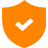The high level of protection is provided by obfuscation both the source code and the binary code.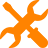The obfuscator supports more than 30 obfuscation methods. Most all of the methods can be enabled, disabled and adjusted independently, with the help of the configuration file. The most effective are:conversion of C++ code into virtual machine code;encryption of strings and arrays;code conversion into a state machine;insertion of dummy links;code section merging.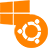The support of all popular operation systems: Windows, macOS, Linux, iOS, Android.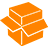StarForce C++ Obfuscator is a stand-alone application that is installed at the customer’s site.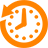Flexible licensing policy (with or without time limitation).An option to set different obfuscation rules for different parts of code.Run from the command line.

## Game protection from tampering and malicious reverse engineering### Situation

Free-to-play games monetization is based on content/resources/artifacts selling. Gamers can use different fraudulent tools (cheats, bots) to get what they want for free. To create a cheat it is necessary to analyze the game code and how it works. The more cheats are in use the less revenue the game publisher has. It even can cause the closing of the project.

### Solution

StarForce C++ Obfuscator prevents from security attacks such as tampering and malicious reverse engineering. The developer himself determines the level of protection for different parts of the code, not to reduce the performance of the game. As a result of obfuscation, each time a new version of the binary code is obtained, which makes it impossible to use the old cheats.

## Source code protection when it is delivered to the third party### Situation

There are many reasons to protect source code: unique algorithms, piracy threat, a part of the code hiding, etc. In case of failure it causes financial and reputational losses.

### Solution

StarForce C++ Obfuscator allows to hide the logic of your software operation. All your secrets will be securely saved.

## Protection from the loss of standard DRM keys### Situation

Standard DRM systems such as AACS and HDCP are used all over the world to control access to audio and video streams. They provide private keys for each model of the user devices (TV sets, players, consoles, set top boxes). A device key leak leads to pirate content distribution and as a result the discredited key is revoked by the DRM. After that the device stops working and a producer needs to get a new key and pays a lot of money for it. Sometimes, a producer may have a penalty for a device key leak, or its license may be suspended.

### Solution

StarForce C++ Obfuscator provides protection for the software that is responsible for video/audio streams delivery and use. After obfuscation the code that is working with DRM keys is reliably protected against analysis and reverse engineering.## Protection of a standard DRM client side

### Situation

The front end of any DRM system, for example OMA DRM, is vulnerable to the attacks that include reverse engineering, modification and protection disabling. Depending on the DRM, an attack can result in compromising either a certain protected object or all of the protected objects of the user, or all of the protected objects in the system.

### Solution

StarForce C++ Obfuscator allows reducing the risk of DRM cracking due to significant increase of the reverse engineering complexity. How the obfuscator should be used (i.e. what should be obfuscated and how) depends on the system’s parameters. To get the optimal solution, please, consult StarForce technical team.## Securing the implementation of custom DRM from reverse engineering

### Situation

The development of custom DRM is a costly task. If the developer decides to do it, he should in any case solve the problem of securing the DRM code that works on the end user side, from reverse engineering. The developer faces two problems in this case:Man-hours for creating an efficient protection against reverse engineering are significant.There is a risk that protection against reverse engineering may not be efficient enough.

### Solution

StarForce C++ Obfuscator enables the developers to save time for the development of a system that protects DRM against reverse engineering. Using StarForce C++ Obfuscator provides a high efficiency level that is known in advance. The developer estimates the level of efficiency when he decides to buy the obfuscator on the basis of the analysis of the obfuscation results.

StarForce C++ Obfuscator is a stand-alone application protected from copying with the help of StarForce ProActive for Business.

For obfuscation unprotected CPP files are used. In the files the methods with the code that should be protected against reverse engineering are marked with special attributes. The result of the obfuscation is also CPP files but the selected methods in them are obfuscated.

## How to use StarForce C++ Obfuscator

 1 Install the obfuscator. No special operations are required. Just unpack the archive with the obfuscator to any folder on a developer’s computer. 2 Activate the obfuscator. Run the omniform.exe executable file without any parameters, enter the serial number and activate the product. 3 Perform test obfuscation. To do that please: А Create the test_input.cpp file with the following contents. __attribute__((obfuscate(0))) int f1( int a, int b ) { if( a > b ) return a; else return b; } B Run the obfuscator with the obfrun.exe test_input.cpp test_output.cpp command. C Make sure that the test_output.cpp file contains the obfuscated code. 4 Prepare the source files for the obfuscation by adding the obfuscate attribute before the required methods. The simplest way to prepare the files is described above; see user guide for details on how to prepare the files. 5 Customize the obfuscator to work with the required compiler by editing the configuration files. 6 Run the obfuscation using command obfrun.exe. 7 Compile the obfuscated files. 7 Test the compiled application and make sure its functionality is equivalent to the one from non-obfuscated files.

To make the obfuscator easier to use, it should be integrated into the application build process during the development.

## The obfuscator can work on the following operating systemsWindows 7 32/64-bitWindows Vista 32/64-bitWindows 8 32/64-bitWindows 10 32/64-bitWindows Server 32/64-bitLinux (option)macOS (Wine)

## The obfuscator requires the following to operate350 Mb of disk space for installation4 GB of RAM

## The obfuscator has the following C++ compatibility parameters

### Supported compilersMSVC6.0, MSVC7.0, MSVC7.1+GCC 3GCC 4

### Supported standarts of C++ ISO/IECC++98C++03C++11C++14C++17

### Supported target platforms for C++WindowsLinuxAndroidmacOSiOS

### Obfuscation of the following C++ language constructions are not supportedMethods that contain Structured Exception Handling (SEH)Constructors and destructorsFunction templates and methods of the template classesSome other structures that are used less often than those mentioned above. The full list of the restrictions is in the user guide# News

16.02.2023
We have released a new version of StarForce Reader for Android
19.01.2023
We have released a new version of StarForce Reader for iOS
13.01.2023
We have released a new version of StarForce Reader for macOS
26.12.2022
We have released a new version of StarForce Reader for Windows

﻿